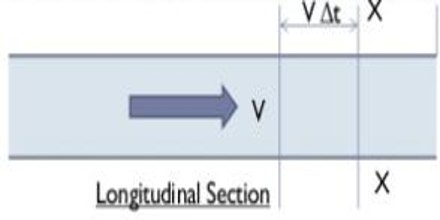# Mean Velocity

Mean velocity: If the direction of motion of a body remains same, the mean value of the initial and final velocities is called mean velocity.

Suppose, initial velocity of a body in a particular direction is v0 and final velocity is v.

So, Mean Velocity = (v0 – v)/2

The possibility to derive an equation for the mean velocity field in turbulent flow by using classical statistical mechanics is investigated. An application of projection operator technique available in the literature is used for this purpose.

Example:

Let’s consider a fluid flowing with mean velocity, V, in a pipe of uniform cross-section. Thus volume of fluid that passes through section XX in unit time ∆t, becomes;Volume of fluid = (∆tV)A

Volume flow rate, Q = volume of fluid/time

= (∆tV)A/∆t